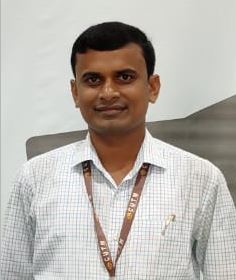Digital Communication SystemsTeacher

Dr. Harish Chandra MohantaCategory

Course Attendees

Still no participant

Still no reviews

Code : CUTM1044 (2-1-0)

Course Objectives

• To impart the fundamentals of modern digital communication system design.
• To evaluate the performance of digital signaling schemes on realistic communication channels.
• Know the techniques of digital communication, information theory, and error control coding.

Learning Outcomes

Upon successful completion of this subject students should be able to:

• Analyze the performance of the baseband and passband digital communication system in terms of error rate and spectral efficiency.
• Perform the time and frequency domain analysis of the signals in a digital communication system.
• Develop an understanding of the performance of digital communication systems.
• Calculate bandwidth and power requirements for digital systems.

Course Syllabus

Module I (05 Hours)

Digital Representation of Analog Signal:

Sampling Theorem, Signal reconstruction from uniform samples, Quantization of signals, Quantization error, PCM, Electrical representation of binary digits, PCM System, Companding.

Practice: (using Trainer Kit and MATLAB)

Analysis of Sampling theorem by using the Trainer Kit and MATLAB.

PCM Modulation and Demodulation using Trainer Kit and MATLAB.

Module II (5 Hours)

Issues in Digital transmission: Line coding, Scrambling, T1-Digital System, Multiplexing T1 lines – The T2, T3, T4, E1 lines, Differential PCM: Linear predicted design, Delta Modulation, Adaptive Delta Modulation.

Practice: (using Trainer Kit and MATLAB)

Differential PCM Modulation and Demodulation using Trainer Kit and MATLAB.

Delta Modulation and Demodulation using Trainer Kit and MATLAB.

Module III (6 Hours)

Digital Modulation Techniques:

Generation, Transmission, Reception, Spectrum, and Geometrical Representation in the Signal Space of BPSK, QPSK, QASK, M-ary PSK, BFSK, M-ary FSK, Minimum Shifting Keying (MSK), QAM, 4-QAM, 8-QAM, and 16-QAM.

Practice : ( using Trainer Kit and MATLAB)

PSK Modulation and Demodulation using Trainer Kit and MATLAB.

FSK Modulation and Demodulation using Trainer Kit and MATLAB.

ASK Modulation and Demodulation using Trainer Kit and MATLAB.

Module IV (5 Hours)

Noise in PCM and DM: Calculation of Quantization Noise, Output Signal Power, and the Thermal Noise, Output SNR in PCM, Quantization noise in Delta Modulation, output signal power, output SNR, Comparison with PCM and DM.

Practice: (using MATLAB)

SNR study of PCM using MATLAB.

SNR study of Delta Modulation using MATLAB.

Module V (3 Hours)

Principle of Digital Data Transmission:

Digital Communication Systems – Source, Line coder, Multiplexer, Regenerative repeater, Line Coding: PSD of various line codes, polar signaling, constructing a DC Null in PSD by pulse shaping, On-Off signaling, Bipolar signaling; Pulse shaping – ISI and effect, Nyquist first criterion for zero ISI, Equalizers, Timing extraction, Detection error, Eye Diagram.

Module VI (5 Hours)

A baseband signal Receiver, Peak signal to RMS noise output voltage ratio, probability of error, optimum threshold, optimum receiver for both baseband and passband: calculation of optimum filter transfer function, optimum filter realization using a Matched filter.

Practice: (using MATLAB)

Study of optimum filter realization using MATLAB.

Module VII (04 Hours)

Discrete Messages and information contents:

Information theory: entropy, mutual information, and channel capacity theorem. Shannon coding, Hoffman coding. Fundamentals of error correction, hamming codes.

Practice: (using MATLAB)

Study of Hamming codes using MATLAB.

Study of error correction using MATLAB.

Reference

Text Book:

Principles of Communication Systems by H Taub, D. L. Schilling, and G Saha, 3rdEdition 2008, TMH Education Pvt. Ltd, New Delhi,

Reference Books:

1. Modern Digital and Analogue Communication Systems by B.P. Lathi and Z Ding, 4th Edition 2010, Oxford University Press, New Delhi.
2. Communication Systems by Simon Haykin, 4thEdition, John Wiley & Sons, Inc.
3. Digital Communications, Proakis, and Salehi, 5th Edition, Pearson Edu.

Session 1

Block diagram of the digital communication system. Advantages and disadvantages of the digital communication system. Sampling Theorem, Signal reconstruction from uniform samples.

DCS-Block Diagram and Sampling Theorem

Session 5

PCM Examples

Lab Practice

PCM Modulation and Demodulation using Trainer Kit and MATLAB.

PCM Experiment

Session 7

T1 Digital System, Multiplexing T1 lines – The T2, T3, T4, E1 lines.

Session 8

Differential PCM: Linear predicted design.

DCS_PPT

Session 9

Differential PCM Modulation and Demodulation.

Lab Practice

Differential PCM Modulation and Demodulation using Trainer Kit and MATLAB.

Session 10

Delta modulation and adaptive delta modulation.

Lab Practice

Delta Modulation and Demodulation using Trainer Kit and MATLAB

PPTs Pdf :

Session 12

Generation, Transmission, Reception, Spectrum, and Geometrical Representation in the Signal Space of M-ary PSK, BFSK.

Session 13

M-ary FSK, and Minimum Shifting Keying (MSK).

DCS_MSK

Session 14

PSK Modulation and Demodulation.

Lab Practice

PSK Modulation and Demodulation using Trainer Kit and MATLAB.

http://vlab.amrita.edu/index.php?sub=59&brch=163&sim=263&cnt=2407

Session 15

Lab Practice

ASK Modulation and Demodulation using Trainer Kit and MATLAB.

Session 16

FSK Modulation and Demodulation.

Lab Practice

FSK Modulation and Demodulation using Trainer Kit and MATLAB.

Session 19

Quantization noise in Delta Modulation, output signal power, output SNR, Comparison with PCM and DM.

Session 20

SNR of PCM

Lab Practice

SNR study of PCM using MATLAB.

Session 21

SNR of delta modulation.

Lab Practice

SNR study of DM using MATLAB

Session 24

Pulse shaping – ISI and effect, Nyquist first criterion for zero ISI.

PPTs Pdf:

Inter Symbol Interference (ISI)

Session 25

Regenerative repeaters, Equalizers, Timing extraction, Detection error, Eye Diagram.

DCS_EYE PATTERN

Session 27

Probability of error, optimum threshold, optimum receiver for both baseband and passband: calculation of optimum filter transfer function.

Session 29

Correlator / Coherent Receiver for a digital carrier modulation.

Lab Practice

Study of optimum filter realization using MATLAB.

Session 33

Hamming code error detection and error detection.

Lab Practice

• Study of Hamming codes using MATLAB.
• Study of error correction using MATLAB.